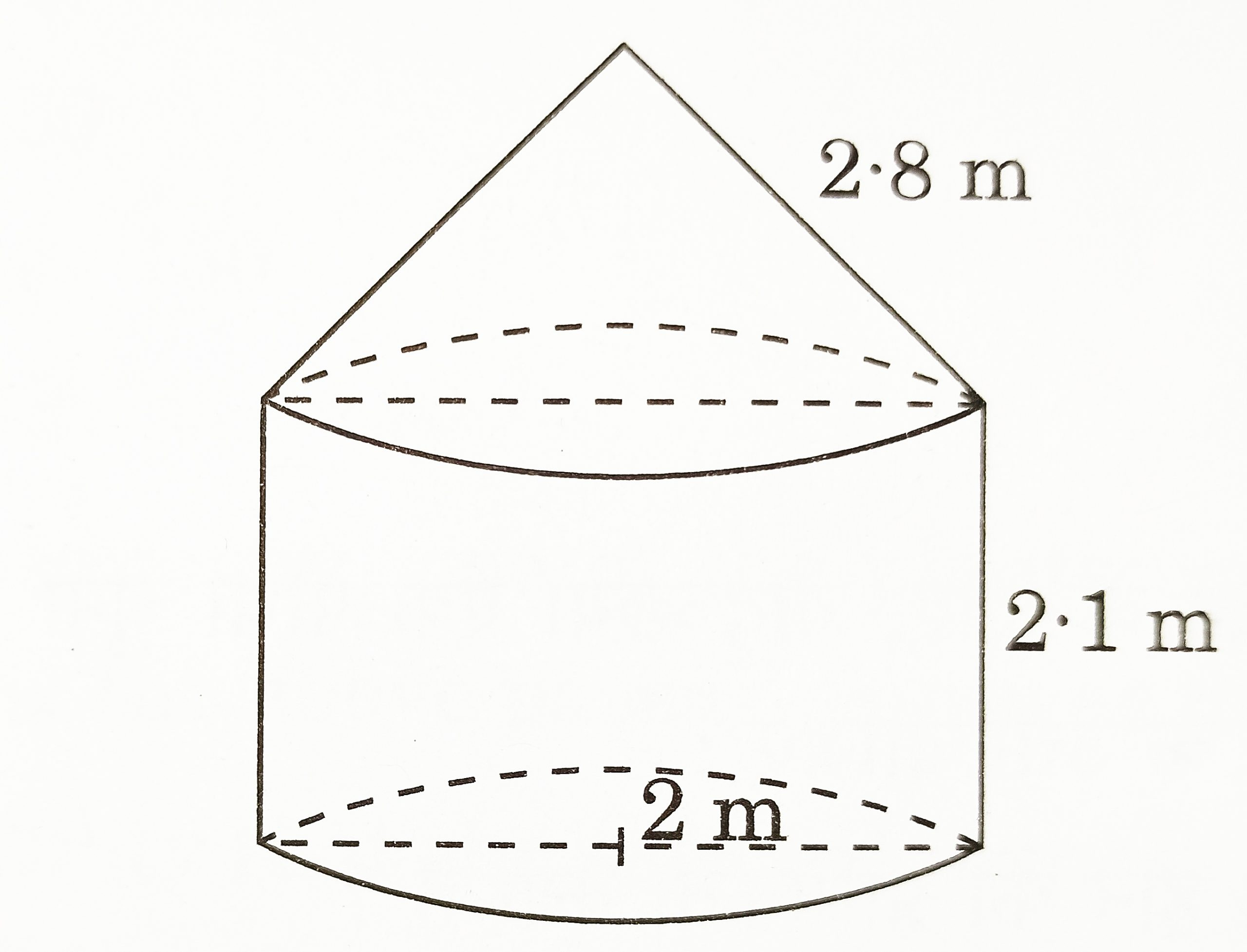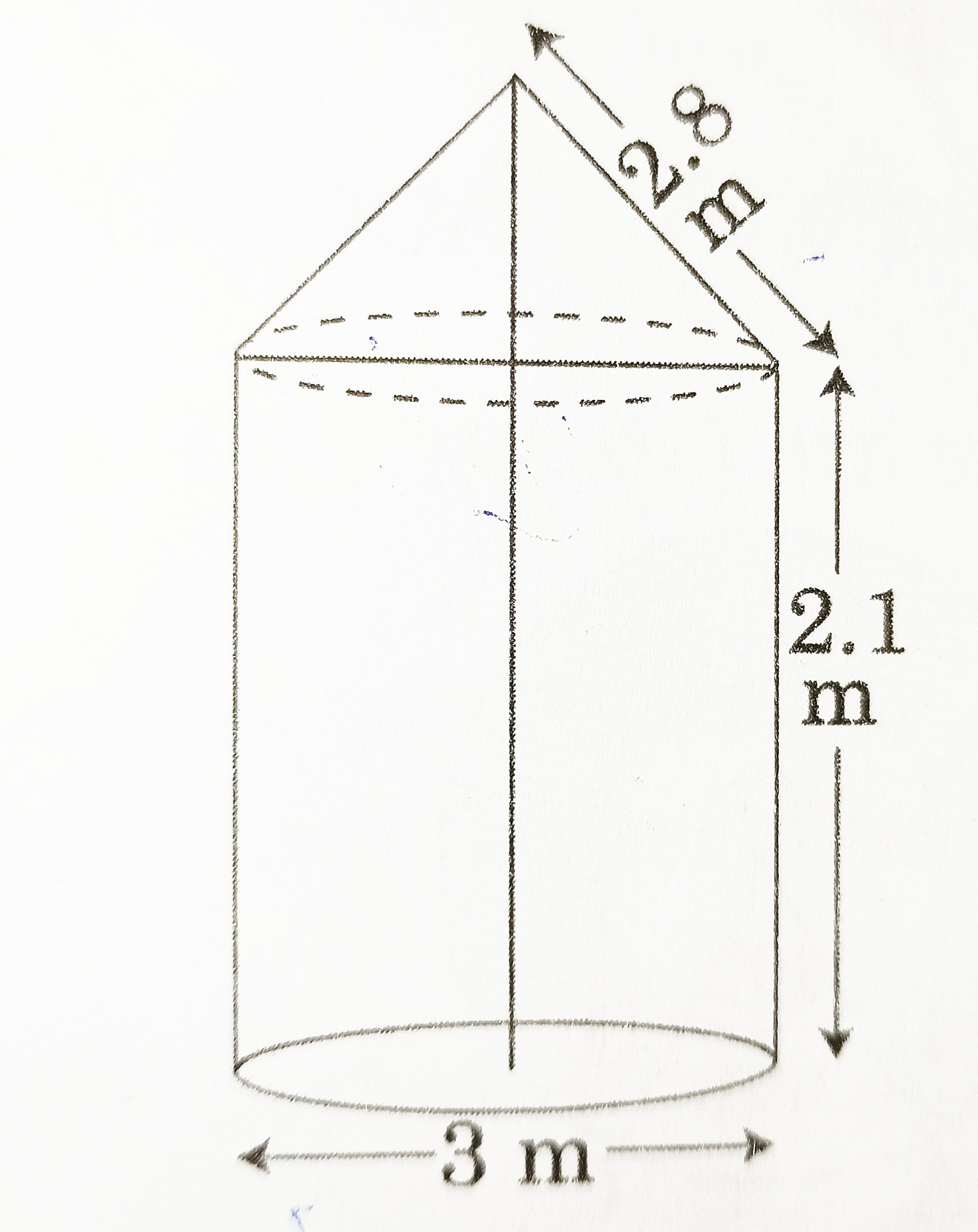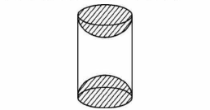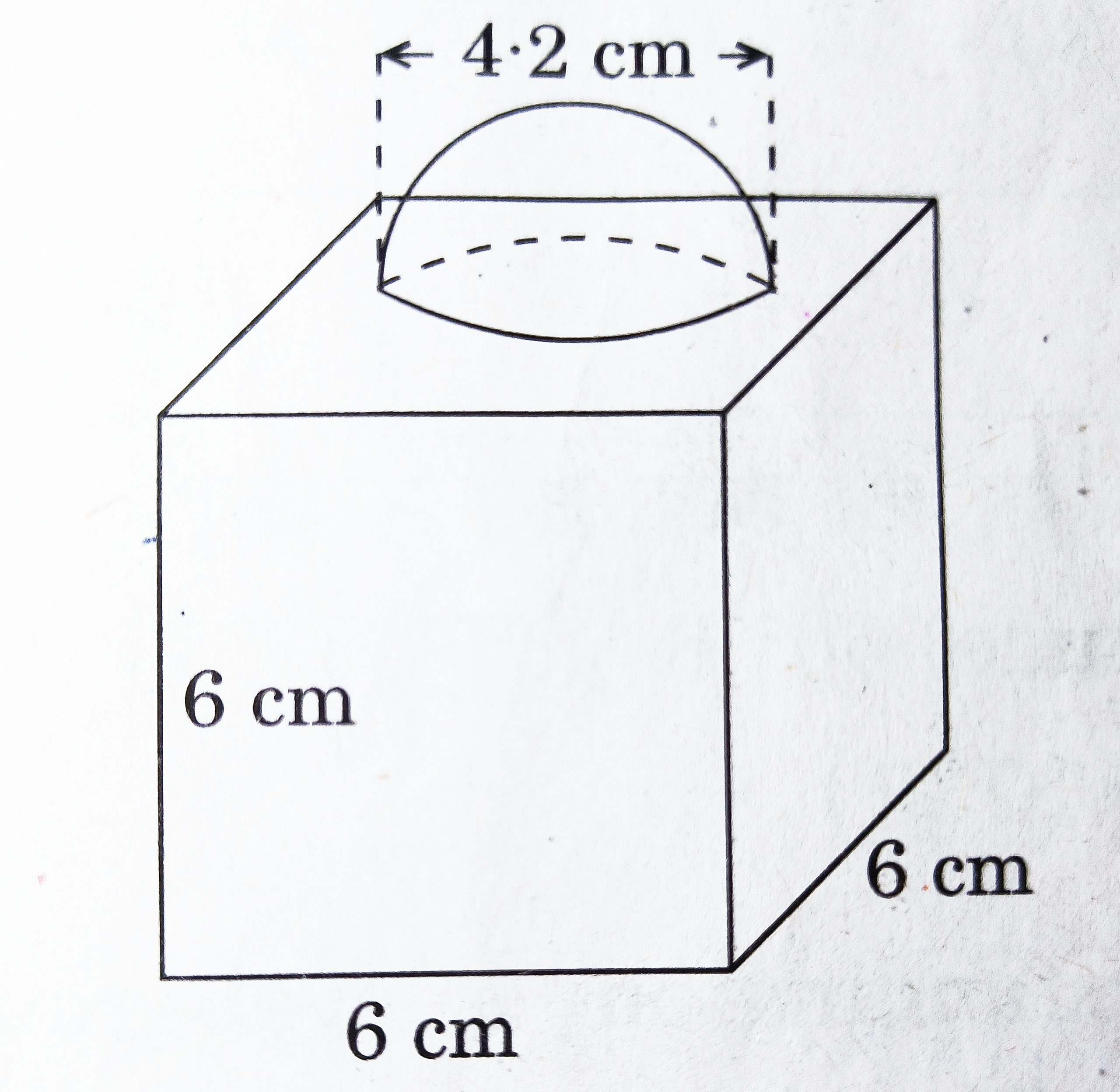# Class 10 – Surface Areas And Volumes – Previous Years Questions

Surface Areas And Volumes – Previous Years Questions

1. A spherical metal ball of radius 8 cm is melted to make 8 smaller identical balls. The radius of each new ball is __________cm. [CBSE 2020] [1 Mark]
2. Find the perimeter of a protector if its diameter is 21 cm. (Use π = 22/7) [CBSE 2017] [2 Marks]
3. In the given figure, a tent is in the shape of a cylinder surmounted by a conical top. The cylindrical part is 2.1 m high and conical part has slant height 2.8 m. Both the parts have same radius 2 m. Find the area of the canvas used to make the tent. (Take π = 22/7) [CBSE 2020] [2 Marks]4. In the given figure, a tent is in the shape of a cylinder surmounted by a conical top of same diameter. If the height and diameter of cylindrical part are 2.1 m and 3 m respectively and the slant height of conical part is 2.8 m. Find the cost of canvas needed to make the tent if the canvas is available at the rate of Rs 500 /sq. meter. (Use π = 22/7)[CBSE 2016] [3 Marks]
5. A conical vessel, with base radius 5 cm and height 24 cm, is full of water. This water is emptied into a cylindrical vessel of base radius 10 cm. Find the height to which the water will rise in the cylindrical vessel. (Use π = 22/7) [CBSE 2016] [3 Marks]
6. A sphere of diameter 12 cm, is dropped in a right circular cylindrical vessel, partly filled with water. If the sphere is completely submerged in water, the water level in the cylindrical vessel rises by cm. Find the diameter of the cylindrical vessel. [CBSE 2016] [3 Marks]
7. A cube of 3 x 3 x 3 units is painted with red colour on all six faces and then it is cut into 27 small equal cubes of 1 x 1 x 1 unit each. A cube is taken out at random. What is the probability that the cube is painted on three sides? [CBSE 2017] [3 marks]
8. A hollow cylindrical pipe is made up of copper. It is 21 dm long. The outer and inner diameters of the pipe are 10 cm and 6 cm respectively. Find the volume of copper used in making the pipe. [CBSE 2017] [3 marks]
9. A warehouse is used as a grannary. It is in the shape of a cuboid surmounted by half cylinder. The base of the warehouse is 6 m x 14 m and its height is 8 m. Find the surface area of the non-cuboidal part of the warehouse. [CBSE 2017] [3 marks]
10. A wooden article was made by scooping out a hemisphere from each end of a solid cylinder, as shown in figure. If the height of the cylinder is 10 cm and its base is of radius 3.5 cm. Find the total surface area of the article. [CBSE 2018] [3 marks]11. A heap of rice is in the shape of a cone of base diameter 24 m and height 3.5 m. Find the volume of the rice. How much canvas cloth is required to just cover the heap ? [CBSE 2018] [3 marks]
12. Water in a canal, 6 m wide and 1.5 m deep, is flowing with a speed of 10 km/h. How much area will it irrigate in 30 minutes if 8 cm of standing water is needed ? [CBSE 2019] [3 marks]
13. Water is flowing at the rate of 2.52 km/h through a cylindrical pipe into a cylindrical tank, the radius of whose base is 40 cm. If the increase in the level of water in the tank, in half an hour is 3.15 m, find the internal diameter of the pipe. [CBSE 2019] [3 marks]
14. Due to heavy flood in a state, thousands were rendered homeless. 50 schools collectively offered to the state government to provide place and the canvas for 1500 tents to be fixed by the government and decided to share the whole expenditure equally. The lower part of each tent is cylindrical of base radius but of height 2.8 m and height 3.5 m, with conical upper part of same base radius but of height 2.1 m. If the canvas used to make the tents costs Rs 120 per sq.m, find the amount shared by each school to set up the tents. What value is generated by the above problem? (Use π = 22/7) [CBSE 2016] [4 Marks]
15. A solid is in the form of a right circular cone mounted on a hemisphere. The radius of the hemisphere is 4.2 cm and height of the cone is 8 cm. The solid is placed in a cylindrical tub, full of water to submerge the whole solid in water. If the radius of cylinder is 10 cm and its height is 19.6 cm, find the volume of water left in the cylindrical tub. (Use π = 22/7) [CBSE 2017] [4 Marks]
16. A farmer wants to dig a well either in the form of cuboid of dimensions 1 m x 1 m x 7 m or in the form of a cylinder of diameter 1 metre and height 7 m. The rate to dig the well is Rs 50 m per cube. Find the cost to dig both wells. The farmer decides to dig the cylindrical well. Why he has chosen cylindrical well ? [CBSE 2017 ] [4 Marks]
17. The diameters of the lower and upper ends of a bucket in the form of a frustum of a cone are 10 cm and 30 cm respectively. If its height is 24 cm, find:
(a) The area of the metal sheet used to make the bucket.
(b) Why we should avoid the bucket made by ordinary plastic ? (Use π = 3.14) [CBSE 2018] [4 Marks]
18. In the given figure, a decorative block is shown which is made of two solids, a cube and a hemisphere. The base of the block is a cube with edge 6 cm and the hemisphere fixed on the top has a diameter of 4.2 cm. Find
(a) the total surface area of the block.
(b) the volume of the block formed. (Take π = 22/7)[CBSE 2019] [4 Marks]
19. A bucket open at the top is in the form of a frustum of a cone with a capacity of 12308.8 cm3. The radii of the top an bottom circular ends are 20 cm and 12 cm respectively. Find the height of the bucket and the area of metal sheet used in making the bucket. (Use π = 3.14) [CBSE 2019] [4 Marks]
20. A bucket open at the top has top and bottom radii of circular ends as 40 cm and and 20 cm respectively. Find the volume of the bucket if its depth is 21 cm. Also, find the area of the tin sheet required for making the bucket. (Use π = 22/7) [CBSE 2020] [4 Marks]